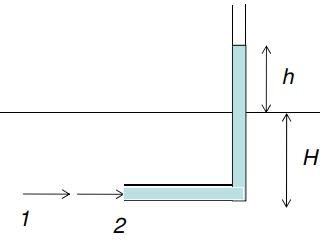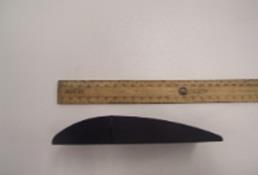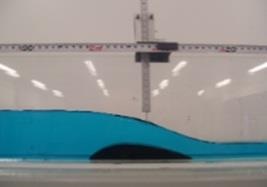### Attain Higher Marks With Our ENGIN1002 Engineering Physics Assignment Help And Assessment Help Service Today!

Home   Course
Previous << || >> Next

ENGIN1002 Engineering Physics, Federation University, Australia

DO YOU WANT TO EXCEL IN ENGIN1002 ENGINEERING PHYSICS ASSIGNMENT - ORDER AT EXPERTSMINDS!

Task: At all data points you can do 2 things:

With dynamic head use Bernoulli to determine the flow velocity at each point. The height of water in the pitot tube relates toi velocity. Since static head is also known at these points, and since the B of the channel is constant (75mm) you know the area of the flow. Use Continuity to determine the mean velocity. u = Q/A. Compare velocities from each method.

The sum of Pressure head + dynamic head + static head should be conserved at all points (within reason). Is it?

Answer: Aim: To determine the flow velocity at each point on a Weir using Bernoulli continuity equation.

Equipment Required: The following equipment is required to carry out the experiment

• Pitot tube
• Scale
• A Pump
• Vernier caliper (for thickness measurement)
• Valve regulators

Formula used:

Bernoulli's continuity equation:

(p/ρg) + (v2/2g) + z = constant

Mean velocity:

u = Q/A

v = √2gh

Description: The main objective of this experiment is to determine the flow velocity at each point on the Weir. The Weir is 150mm long and 75 mm wide. The thickness of the weir is around 25mm. The flow is measured using the pitot tube. The pitot tube is a device used to measure the flow of liquid. It is based on the principle that the pressure at a point increases if the velocity of the fluid at that point decreases. This is a result of the conservation of kinetic energy into potential energy.The pitot tube comprises a glass tube which is bent at right angles. It is in accordance with Bernoulli's equation.

SAVE YOUR HIGHER GRADE WITH ACQUIRING ENGIN1002 ENGINEERING PHYSICS ASSIGNMENT HELP AND QUALITY ASSESSMENT WRITING SERVICES OF EXPERTSMINDS.COM!

Derivation:

Bernoulli's equation states that,

(p/ρ) + gz + (v2/2g) = constant

Multiplying ρ on both sides we get

p + q + ρgz = constant

p + q + ρgz = constant

where q = v2/2g

p+ ρgz = constant

p= p + q

p= sum of static pressure and dynamic pressure

Dividing by ρg we get

(p/ρg) + (v2/2g) + z = constant

Total head H is given by

H = (p/ρg) + (v2/2g) + z

H = (v2/2g) + h

where h = p/ρg + z

Procedure: The dimensions of the weir are measured with the help of a scale. The weir ranges about 150 mm long and 75mm wide. The thickness of the weir is measured using the vernier caliper. The readings indicate that the weir is 25mm thick. The weir is placed in a setup which comprises a pump and a regulator.The image illustrates measuring the weir with the help of a scale

The pump is capable of lifting the liquid through mechanical action. The set up also comprises of a pitot tube. The pitot tube is a device that is used to measure the flow of the fluid. The pitot is generally a glass tube that is bent at right angles. It is based on the principle of Bernoulli's equation.The image illustrates the measurement of dynamic and static heat using a pitot tube

The liquid is allowed to flow over the weir. This flow rate of the liquid is regulated using a valve. Assumptions are made that the left side of the weir i.e the front edge of the weir is the initial point. The upstream to the weir is negative and downstream is considered to be positive. Using the pitot tube the dynamic head at each point is calculated. Similarly, the static head at the same point is also determined. The points are taken from the start of the weir to its end (0<x<150) with respect to its length. The static head is measured from the water surface to the channel floor on either side of the object. The values of the static head and dynamic head at each point are observed and the flow velocity at each point is calculated. Comparisons are made on the basis of the flow velocity with respect to static and dynamic heads.

GET GUARANTEED SATISFACTION OR MONEY BACK UNDER ENGIN1002 ENGINEERING PHYSICS ASSIGNMENT HELP SERVICES OF EXPERTSMINDS.COM - ORDER TODAY NEW COPY OF THIS ASSIGNMENT!

Calculation:

Mean velocity

u = Q/A

Area of the weir is given by= (Length.Breadth)

= 150(75) = 11250mm

Discharge Q = (CD √2gh)/A

Q = (0.8 √2gh)/A

Q = (0.8√(19.62(90)))/11250

Q = 2.988 × 103

U = 2.65m/s

h = (p/ρg) + z

Total head H is given by

H = (p/ρg) + (v2/2g) + z

H = (v2/2g) + h

(p/ρg) + (v2/2g) + z = constant

Before water enters the tube

P = 1 atm v = ? h = 0

At this point

(p/ρg) = (v2/2g)

(1/1000) = (v2/2)

v = 4.54m/s

When the water rises to the top

P = 1 atm v = 0 h = 90

H = (v2/2g) + h

h = (p/ρg) = 10332.279/(1000(9.81)) = 1.053m

v = √(2p/ρ)

V = 4.54m/s

Total head at point 1 (initial)

H = (p/ρg) + (v2/2g)

H = 1.05m

Total head at point 2 (top)

H = (p/ρg) + (v2/2g)

H = 1.05m

Therefore the head at both the points are equal.

It can be clearly seen that as the velocity decreases pressure increases.

Our Federation University, Australia assignment help services are delivered for different other courses and majors like -

• ENGIN1001 - Professional Engineering Assignment Help
• ENGIN1003 - Materials In Engineering Assignment Help
• ENGIN1004 - Engineering Design & Drafting Assignment Help
• ENGIN1005 - Engineering Mechanics Assignment Help
• ENGIN1006 - Engineering Computer Modelling Assignment Help
• ENGIN2001 - Professional Practice Assignment Help
• ENGIN2002 - Engineering Project Management And Sustainable Design Assignment Help
• ENGIN2101 - Digital Logic And Design Assignment Help
• ENGIN2102 - Signals And Systems Assignment Help
• ENGIN2103 - Principles Of Renewable Energy Sources Assignment Help
Tag This :- EM201990KHU531PHY ENGIN1002 Engineering Physics Assignment Help### Assignment Samples

 BSBWOR502 Lead and Manage Team Effectiveness Assignment Help bsbwor502 lead and manage team effectiveness assignment help - Orange International College - Describe four strategies you would implement Evolutions of Health Care Assignment Help evolutions of health care assignment help - Write a 3-4-page paper in which you review how health informatics began, and how it has evolved over the years. Mars Chocolate - Marketing Strategy Analysis Assignment Help mars chocolate - marketing strategy analysis assignment help - how the marketing mix can be used to determine various elements of the product Legal and Ethical Scenarios Assignment Help legal and ethical scenarios assignment help - Two legal and ethical cases discussed. The first is title VII and discrimination case. Psychology Assignment Help psychology assignment help - The present solution is based on the concept of psychology, business consultancy, and various theories of psychology. Importance of National Safety and Quality Health Service Standards in the Australian Health System Assignment Help importance of national safety and quality health service standards in the australian health system assignment help Objectives & Target Market Assignment Help objectives & target market assignment help - The current report has developed SMART objectives and targeting strategies for The Coca Cola Company.Get Academic Excellence with Best Skilled Tutor! Order Assignment Now!